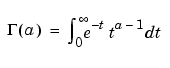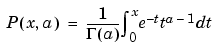MATLAB Function Referencegamma, gammainc, gammaln

Gamma functions

Syntax

• ```Y = gamma(A)                Gamma function
Y = gammainc(X,A)           Incomplete gamma function
Y = gammainc(X,A,tail)      Tail of the incomplete gamma function
Y = gammaln(A)              Logarithm of gamma function
```

Definition

The gamma function is defined by the integral:

•The gamma function interpolates the factorial function. For integer `n`:

• ```gamma(n+1) = n! = prod(1:n)
```

The incomplete gamma function is:For any `a>=0`, `gammainc(x,a)` approaches 1 as `x` approaches `infinity`. For small `x` and `a`, `gammainc(x,a)` is approximately equal to `x^a`, so `gammainc(0,0) = 1`.

Description

```Y = gamma(A) ``` returns the gamma function at the elements of `A`. `A` must be real.

```Y = gammainc(X,A) ``` returns the incomplete gamma function of corresponding elements of `X` and `A`. Arguments `X` and `A` must be real and the same size (or either can be scalar).

`Y = gammainc(X,A,tail)` specifies the tail of the incomplete gamma function when `X` is non-negative. The choices are for `tail` are `'lower'` (the default) and `'upper'`. The upper incomplete gamma function is defined as

• ```1 - gammainc(x,a)
```

 Note    When `X` is negative, `Y` can be inaccurate for `abs(X)>A+1`.

```Y = gammaln(A) ``` returns the logarithm of the gamma function, `gammaln(A) = log(gamma(A))`. The `gammaln` command avoids the underflow and overflow that may occur if it is computed directly using `log(gamma(A))`.

Algorithm

The computations of `gamma` and `gammaln` are based on algorithms outlined in . Several different minimax rational approximations are used depending upon the value of `A`. Computation of the incomplete gamma function is based on the algorithm in .

References

  Cody, J., An Overview of Software Development for Special Functions, Lecture Notes in Mathematics, 506, Numerical Analysis Dundee, G. A. Watson (ed.), Springer Verlag, Berlin, 1976.

  Abramowitz, M. and I.A. Stegun, Handbook of Mathematical Functions, National Bureau of Standards, Applied Math. Series #55, Dover Publications, 1965, sec. 6.5.

© 1994-2005 The MathWorks, Inc.